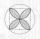# Diameters of circles

How many percent of the area of a larger circle is a smaller circle if the smaller circle has a diameter 120 mm and a larger one has a diameter 300 mm?

Result

p =  16 %

#### Solution:Leave us a comment of example and its solution (i.e. if it is still somewhat unclear...):Be the first to comment!## Next similar examples:

1. Circular lawnAround a circular lawn area is 2 m wide sidewalk. The outer edge of the sidewalk is curb whose width is 2 m. Curbstone and the inner side of the sidewalk together form a concentric circles. Calculate the area of the circular lawn and the result round to 1
2. PersonsPersons surveyed:100 with result: Volleyball=15% Baseball=9% Sepak Takraw=8% Pingpong=8% Basketball=60% Find the average how many like Basketball and Volleyball. Please show your solution.
3. ConfectioneryThe village markets have 5 kinds of sweets, one weighs 31 grams. How many different ways a customer can buy 1.519 kg sweets.
4. ChordsHow many 4-tones chords (chord = at the same time sounding different tones) is possible to play within 7 tones?
5. PIN - codesHow many five-digit PIN - code can we create using the even numbers?
6. QuatrefoilCalculate area of the quatrefoil which is inscribed in a square with side 6 cm.
7. AceThe length of segment AB is 24 cm and the point M and N divided it into thirds. Calculate the circumference and area of this shape.
8. Percentages52 is what percent of 93?
9. Profit gainIf 5% more is gained by selling an article for Rs. 350 than by selling it for Rs. 340, the cost of the article is:
10. BorrowingI borrow 25,000 to 6.9% p.a.. I pay 500 per month. How much will I pay and for how long?55%+36%+88%+71%+100=63% what is whole (X)? Percents can be added directly together if they are taken from the same whole, which means they have the same base amount. .. . You would add the two percentages to find the total amount.52 shorts and missed 13. Calculate percentageWe want to prove the sentence: If the natural number n is divisible by six, then n is divisible by three. From what assumption we started?Solve the system of linear equations with three unknowns: A + B + C = 14 B - A - C = 4 2A - B + C = 0Write the first 5 members of an arithmetic sequence a11=-14, d=-1It is true that the lines that do not intersect are parallel?Determine the first nine elements of sequence if a10 = -1 and d = 4